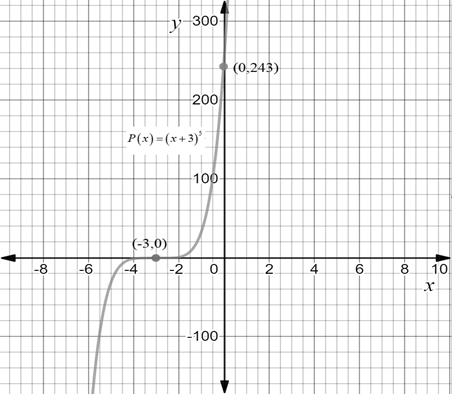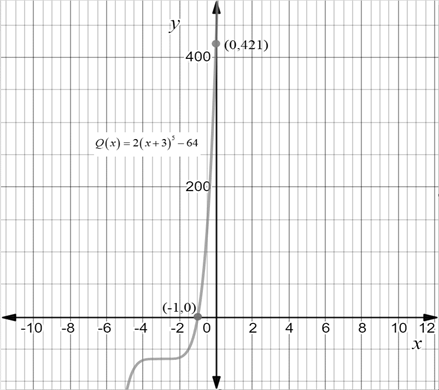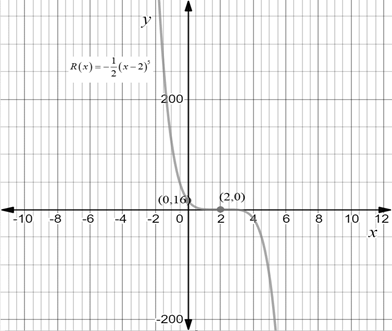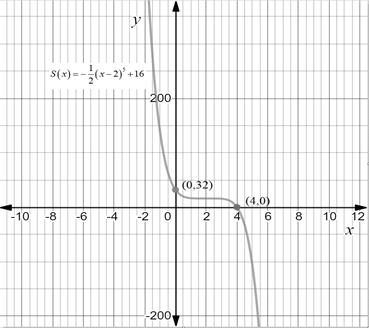# The graph of the function P ( x ) = ( x + 3 ) 5 by transforming the graph of an appropriate function of the form y = x n and indicate all x and y intercepts on graph.### Precalculus: Mathematics for Calcu...

6th Edition
Stewart + 5 others
Publisher: Cengage Learning
ISBN: 9780840068071### Precalculus: Mathematics for Calcu...

6th Edition
Stewart + 5 others
Publisher: Cengage Learning
ISBN: 9780840068071

#### Solutions

Chapter 3.2, Problem 8E

(a)

To determine

## To sketch: The graph of the function P(x)=(x+3)5 by transforming the graph of an appropriate function of the form y=xn and indicate all x and y intercepts on graph.

Expert Solution

### Explanation of Solution

Given information:

The given functions is P(x)=(x+3)5 .

Graph:

The graph of the functions P(x)=(x+3)5 is as follows.Interpretation:

The graph of P(x)=(x+3)5 is the graph of y=x5 which is shifted left 3 units.

(b)

To determine

Expert Solution

### Explanation of Solution

Given information:

The given functions is Q(x)=2(x+3)564 .

Graph:

The graph of the functions Q(x)=2(x+3)564 is as follows.Interpretation:

The graph of Q(x)=2(x+3)564 is the graph of y=x5 which is shifted to the left 3 units and then shifted downward 64 units.

(c)

To determine

Expert Solution

### Explanation of Solution

Given information:

The given functions is R(x)=12(x2)5 .

Graph:

The graph of the functions R(x)=12(x2)5 is as follows.Interpretation:

The graph of R(x)=12(x2)5 is the graph of y=x5 which is shifted to the right 2 units.

(d)

To determine

Expert Solution

### Explanation of Solution

Given information:

The given functions is S(x)=12(x2)5+16 .

Graph:

The graph of the functions S(x)=12(x2)5+16 is as follows.Interpretation:

The graph of S(x)=12(x2)5+16 is the graph of y=x5 which is shifted to the right 2 units and then shifted upward 16 units.

### Have a homework question?

Subscribe to bartleby learn! Ask subject matter experts 30 homework questions each month. Plus, you’ll have access to millions of step-by-step textbook answers!